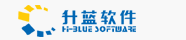# 利用MSCHART画图的一段代码

March 25,2004
<!-- Here we are calling the LPK file.  This LPK file
was created using the same method explained in the article-->

<object CLASSID="clsid:5220cb21-c88d-11cf-b347-00aa00a28331"
<param NAME="LPKPath" VALUE="mschart5.lpk">
</object>

<-- Here is where we are actually "instantiating" the
Microsoft's website.  It has been digitally signed. -->

<OBJECT ID="MSChart1" WIDTH=100% HEIGHT=95%
CLASSID="CLSID:31291E80-728C-11CF-93D5-0020AF99504A"
codebase="mschart5.cab">
</OBJECT>

<script LANGUAGE="Vbscript">
'Now, let's set the chart properties...!!!

'This sets the chart to a line graph...
MSChart1.ChartType = 3

'Set the color and width of the line
' This sets the pen color to black (0,0,0)
' and width to 50 pixels.
MSChart1.Plot.SeriesCollection(1).Pen.VtColor.Set 0,0,0
MSChart1.Plot.SeriesCollection(1).Pen.Width = 50

'This sets the chart's labels to various formats,
' fonts, and sizes.
For i = 1 To MSChart1.Plot.Axis(1).Labels.Count
'Format the Chart labels to Currency
MSChart1.Plot.Axis(1).Labels(i).Format = "\$0,###"

'Set the font to Tahoma
MSChart1.Plot.Axis(1).Labels(i).VtFont.Name = "Tahoma"

'Set the font size to 10pt
MSChart1.Plot.Axis(1).Labels(i).VtFont.Size = 10
Next

'This sets the # of rows in the chart
MSChart1.RowCount = 5;

'This sets the number of columns per row.
MSChart1.ColumnCount = 2

'This indicates to show the label
MSChart1.ShowLegend = True

for x = 1 to 5  'The number of rows we have
'This sets what current row we are editing
MSChart1.Row = x

'This sets the row's label
MSChart1.RowLabel = "Row " & x

'This plots the points for both columns (1 and 2)
'for the current row (x).  The value being
'plotted is x*5 and x*10
call MSChart1.DataGrid.SetData(x, 1, x*5,nullFlag)
call MSChart1.DataGrid.SetData(x, 2, x*10,nullFlag)
next

</script>